## 量化分类

### 对称量化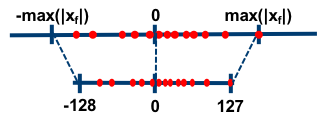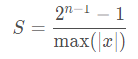### 非对称量化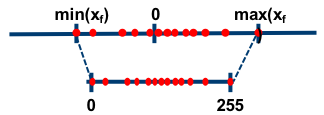在非对称量化中，r 是用有符号的整型数值(uint8)来表示的。在非对称量化中，我们可以取Z=min(x)，S的取值可以使用如下的公式，也可以采用其它的公式。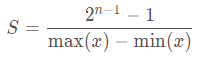## 原理详解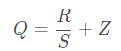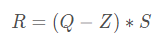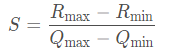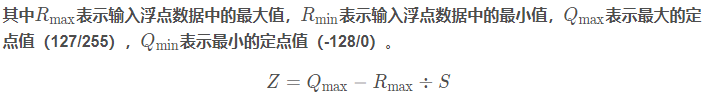### 具体案例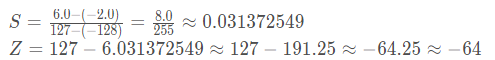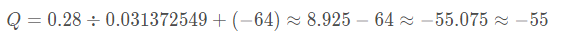## tensorflow量化分类

### 训练后的量化

tensorflow训练后量化是针对已训练好的模型而言的，针对大部分我们已训练好的的网络模型来说均可使用此方法进行模型量化。tensorflow提供了一整套完整的模型量化工具，如TensorFlow Lite Optimizing COnverter（toco命令工具）以及TensorFlow Lite converter（API源码调用接口）。

#### 混合量化-仅量化权重

``````import tensorflow as tf
# 装载预训练模型
converter = tf.lite.TFLiteConverter.from_saved_model(saved_model_dir)
# 设置优化器
converter.optimizations = [tf.lite.Optimize.OPTIMIZE_FOR_SIZE]
# 执行转换操作
tflite_quant_model = converter.convert()
``````

#### 全整型量化-权重和激活值都进行量化

全整型量化的输入输出依然是浮点型的，但如果某些Ops未实现该方法，则转化是没问题的且其依然会自动保存为浮点型，这就要求我们的硬件支持这样的操作。

``````import tensorflow as tf

def representative_dataset_gen():
for _ in range(num_calibration_steps):
# Get sample input data as a numpy array in a method of your choosing.
yield [input]

# 装载预训练模型
converter = tf.lite.TFLiteConverter.from_saved_model(saved_model_dir)
# 设置优化器
converter.optimizations = [tf.lite.Optimize.DEFAULT]
# 获得标注数据
converter.representative_dataset = representative_dataset_gen
# 执行转化操作
tflite_quant_model = converter.convert()
``````

#### 半精度量化-仅量化权重

``````import tensorflow as tf
# 装载预训练模型
converter = tf.lite.TFLiteConverter.from_saved_model(saved_model_dir)
# 设置优化器
converter.optimizations = [tf.lite.Optimize.DEFAULT]
# 判断当前的设备是否支持FP16操作
converter.target_spec.supported_types = [tf.lite.constants.FLOAT16]
# 执行转换
tflite_quant_model = converter.convert()
``````

## 量化具体实现

### 训练后的量化

``````def tflite_from_sess(ckpt_dir, out_path):
net = Det(ckpt_dir)
with net.sess as sess:
converter = tf.lite.TFLiteConverter.from_session(sess, [net.input_images], [net.seg_maps_pred])
converter.post_training_quantize = True   # 启用训练后模型量化，进行量化转换，模型大小会小很多
tflite_model = converter.convert()
open(out_path, "wb").write(tflite_model)
``````

### 训练中引入量化(量化感知训练)

tensorflow中的训练时量化是一种伪量化。它是在可识别的某些操作内嵌入伪量化节点（fake quantization nodes），用以统计训练时流经该节点数据的最大值和最小值便于在使用TOCO转换tflite格式时量化使用并减少精度损失，它参与模型训练的前向推理过程令模型获得量化损失，但梯度更新需要在浮点下进行因而其并不参与反向传播过程。某些操作无法添加伪量化节点，这时候就需要人为的去统计某些操作的最大最小值，但如果统计不准确那么将会带来较大的精度损失，因而需要谨慎检查哪些操作无法添加伪量化节点。值得注意的是，伪量化节点的意义在于统计流经数据的最大最小值并参与前向传播过程来提升模型精度，但其在TOCO工具转换为量化模型后，其工作原理还是与训练后量化方式一致的！具体的量化流程如下图所示：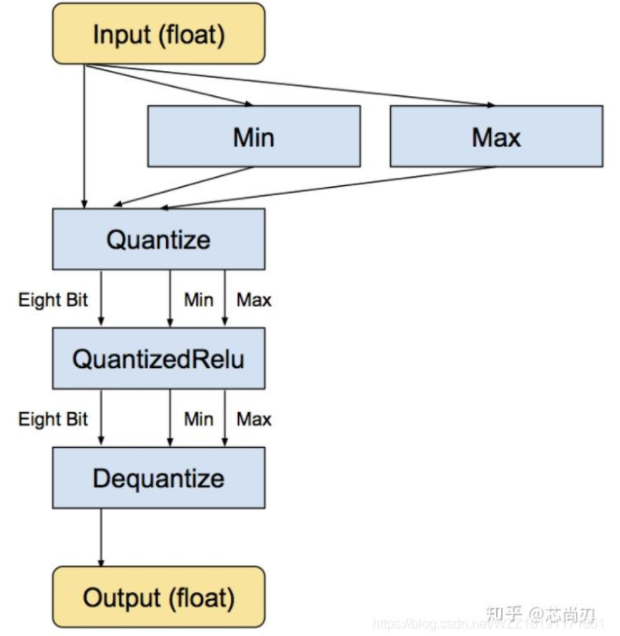#### 实战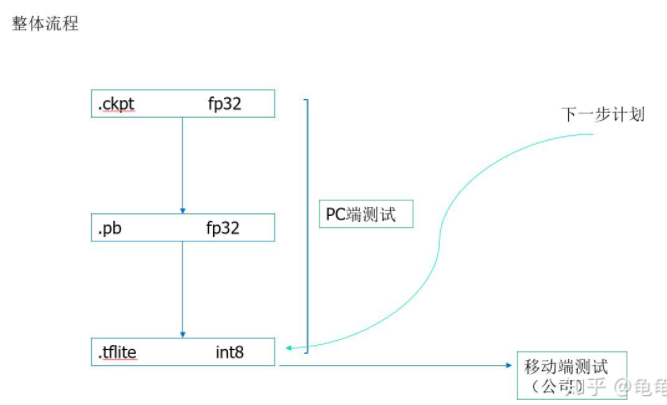##### 步骤1-在训练图结构内添加伪量化节点

``````# 获取loss函数
loss = tf.losses.get_total_loss()
# 获取原始的图，并在原始的图的基础上创建一个量化图
g = tf.get_default_graph()
tf.contrib.quantize.create_training_graph(input_graph=g, quant_delay=2000000)
# 设计优化器并执行反向传播
optimizer.minimize(loss)
``````

tf.contrib.quantize.create_training_graph的参数input_graph表示训练的默认图层，quant_delay是指多少次迭代之后再进行量化训练，如果是已训练好进行微调量化的话，那么可以将quant_del ay设为0。
quant_delay表示网络在进行量化训练前采用浮点训练的次数，在源码中，quant_delay默认为0。

##### 步骤2-重写推理图结构并保存为新的模型

``````def saveQuantCkpt2Pb(in_ckpt_dir,output_node_names):
net = MnistNet(False)  # 网络定义在默认图上
graph = tf.compat.v1.get_default_graph()  # 获取默认图
tf.contrib.quantize.create_eval_graph(graph)  # 在默认图基础上创建一个推理图,使其包含量化结点

sess = tf.compat.v1.Session(graph=graph)  # 创建一个会话，设置会话的图为graph

# 创建一个模型保存和加载对象
saver = tf.compat.v1.train.Saver()
ckpt = tf.train.latest_checkpoint(in_ckpt_dir)  # 查找in_ckpt_dir目录下最新保存的checkpoint文件的文件名
print("ckpt:================", ckpt)
saver.restore(sess, ckpt)   # 将量化训练的ckpt模型参数加载到当前默认会话

# 同时将网络模型结构与参数用二进制格式的pb文件保存
# sess.graph.as_graph_def()：导出当前计算图的GraphDef部分，GraphDef保存了从输入层到输出层的计算过程
converted_graph_def = tf.compat.v1.graph_util.convert_variables_to_constants(sess, input_graph_def=sess.graph.as_graph_def(),
output_node_names=output_node_names)  # #保存指定的节点，并将节点值保存为常数
# tf.gfile.GFile(filename, mode)：获取文本操作句柄，类似于python提供的文本操作open()函数，filename是要打开的文件名，mode是以何种方式去读写，将会返回一个文本操作句柄。
# tf.gfile.Open()是该接口的同名，可任意使用其中一个
# with tf.io.gfile.GFile(pb_graph_dir, "wb") as f:   # 保存方法一
#     f.write(converted_graph_def.SerializeToString())  # SerializeToString()序列化

tf.io.write_graph(  # 保存方法二
converted_graph_def,
"./pb",
"freeze_eval_graph.pb",
as_text=False)
``````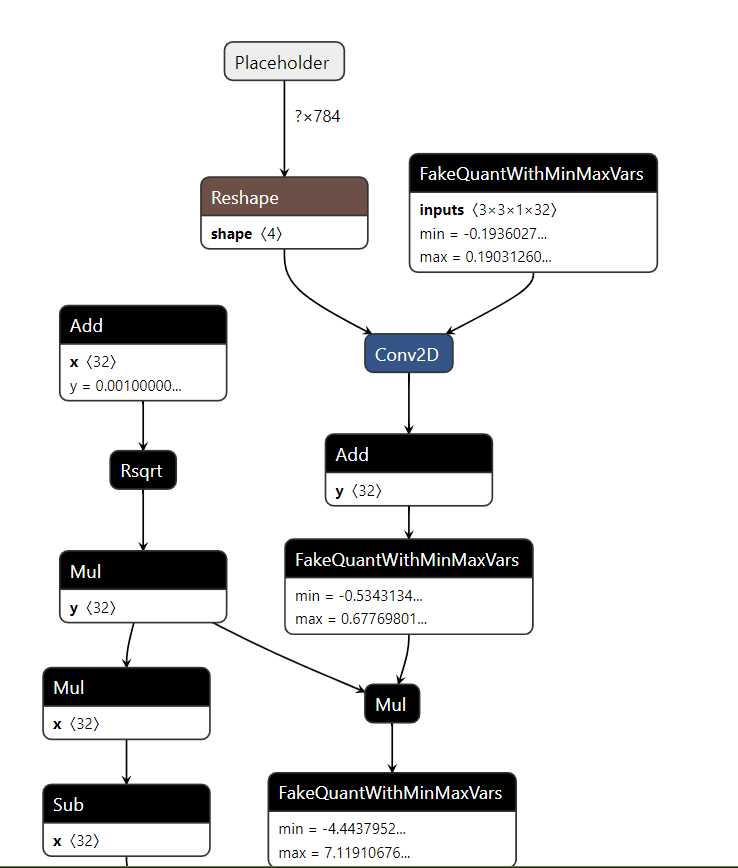##### 步骤3-量化：将pb模型转换成tflite文件

``````# 量化后的pb模型转为tflite

import tensorflow as tf

path_to_frozen_graphdef_pb = './pb/freeze_eval_graph.pb'
input_arrays = ["Placeholder"]
output_arrays = ["Softmax"]
input_shapes = {'Placeholder': [1, 784]}
converter = tf.lite.TFLiteConverter.from_frozen_graph(path_to_frozen_graphdef_pb,
input_arrays,  # 是freeze pb中的输入节点
output_arrays,  # 是freeze pb中的输出节点
input_shapes = input_shapes)

# converter.target_ops = [tf.lite.OpsSet.TFLITE_BUILTINS,tf.lite.OpsSet.SELECT_TF_OPS]
converter.inference_type = tf.uint8      # 标识进行uint8非对称量化
# quantized_input_states是定义输入的均值和方差
converter.quantized_input_stats = {"Placeholder": (127.5, 127.5)}
converter.allow_custom_ops = True
# default_ranges_states是指量化后的值的范围，其中255就是2^8 - 1
converter.default_ranges_stats = (0, 255)  # 由于是进行uint8量化，所以量化值的输出范围为[0,255]
tflite_model = converter.convert()  # 根据pb模型中的最大最小值等标定数据对模型执行量化操作，即将其由FP32转换为INT8
open("./tflite/eval_graph.tflite", "wb").write(tflite_model)
print("finish")
``````

• range (0,255) then mean = 0, std_dev = 1
• range (-1,1) then mean = 127.5, std_dev = 127.5
• range (0,1) then mean = 0, std_dev = 255

``````toco  --graph_def_file
./frozen_inference_graph.pb
--output_file test.tflite
--input_format=TENSORFLOW_GRAPHDEF
--output_format=TFLITE
--inference_type=QUANTIZED_UINT8
--input_shape='1,300,300,3' --input_array='FeatureExtractor/MobilenetV2/MobilenetV2/input' --output_array='concat,concat_1'
--std_dev_value 127.5
--mean_value 127.5
--default_ranges_min 0
--default_ranges_max 255
``````

## tensorflow量化方法比较

• 两者均可达到模型量化的作用；
• 两者的推理工作原理是一样的；
• 两者都可工作在Tensorflow lite推理框架下并进行相应加速；

• 前者是一种offline的方式，而后者则是一种online的方式；
• 训练后量化工作量稍微简单些，而量化感知训练工作量更繁琐一些；
• 量化感知训练比训练后量化损失的精度更少，官方推荐使用量化感知训练方式；